﻿ 基于自编码器的特征迁移算法
«上一篇文章快速检索 高级检索

 智能系统学报2017, Vol. 12Issue (6): 894-898  DOI: 10.11992/tis.2017060370

### 引用本文YANG Mengduo, LUAN Yonghong, LIU Wenjun, et al. Feature transfer algorithm based on an auto-encoder[J]. CAAI Transactions on Intelligent Systems, 2017, 12(6): 894-898. DOI: 10.11992/tis.201706037.### 文章历史

1. 苏州工业职业技术学院 软件与服务外包学院，江苏 苏州 215104;
2. 苏州大学 计算机科学与技术学院，江苏 苏州 215006

Feature transfer algorithm based on an auto-encoder
YANG Mengduo1, LUAN Yonghong1, LIU Wenjun1, LI Fanzhang2
1. Department of Software and Service Outsourcing, Suzhou Vocational Institute of Industrial Technology, Suzhou 215104, China;
2. School of Computer Science and Technology, Soochow University, Suzhou 215006, China
Abstract: The stacked auto-encoder (SAE) has recently shown outstanding image classification performance in large-scale datasets. Relative to the low-level features of artificial design in other image classification methods, the success of SAE is its deep network that can learn rich mid-level image features. However, estimating millions of parameters requires a very large number of annotated image samples, and this prevents many SAE applications to small-scale training data. In this paper, the proposed algorithm shows how to efficiently transfer image representation learned by SAE on a large-scale dataset to other visual recognition tasks with limited training data. In the experimental section, a method is designed to reuse the hidden layers trained on MNIST datasets to compute the mid-level image representation of MNIST-variation datasets. Experimental results show that, despite differences in image datasets, the transferred image features can significantly improve the classification performance of the model.
Key words: auto-encoder    feature transfer    deep network    deep learning    image classification    mid-level image representation    visual recognition    large-scale datasets

1 自编码器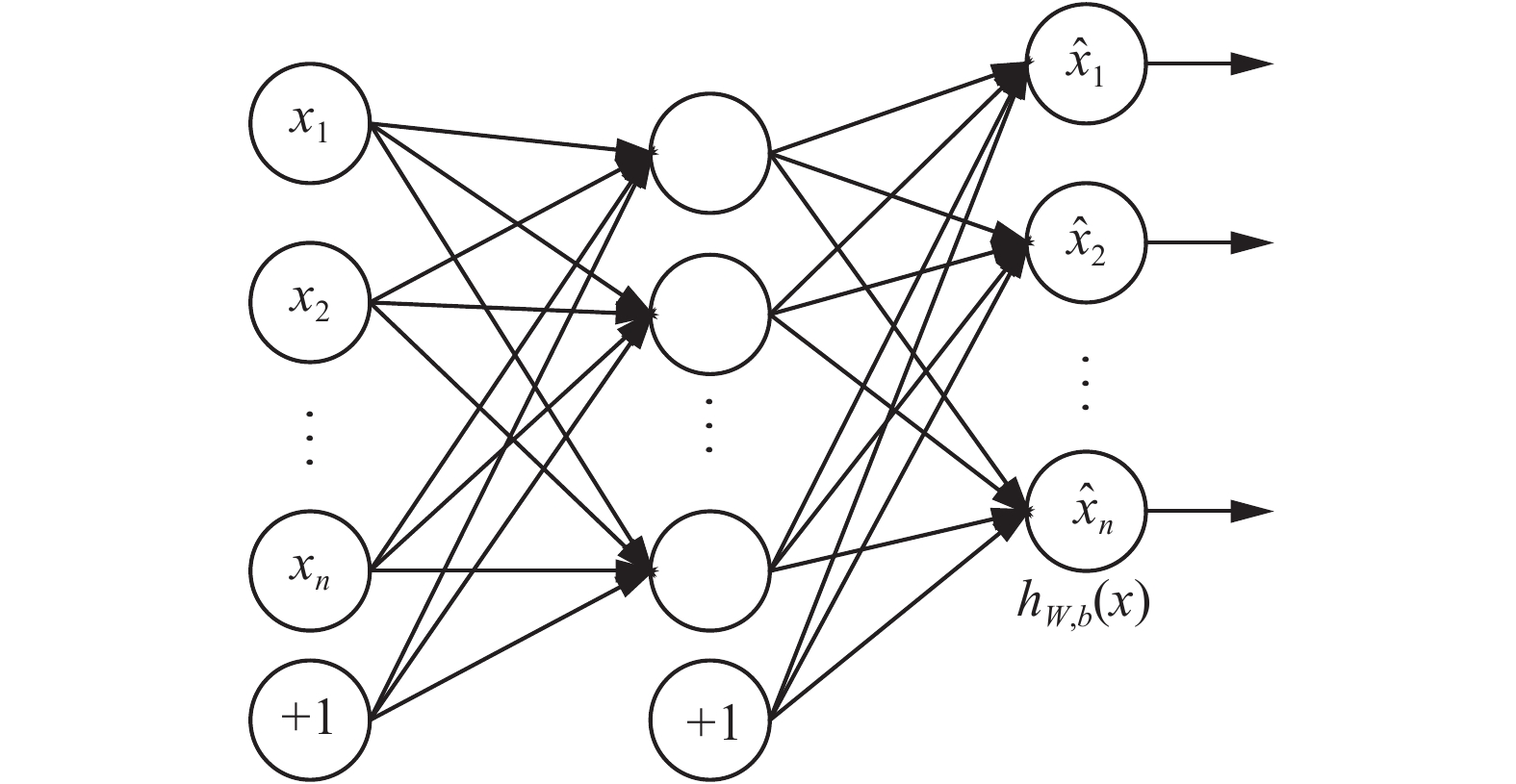图 1 自编码器示意图 Fig.1 Auto-encoder diagram

${h_{{\mathit{\boldsymbol{W}}},{\mathit{\boldsymbol{b}}}}}({\mathit{\boldsymbol{x}}})$ 定义了一个复杂且非线性的函数以对每一个训练样本 ${\mathit{\boldsymbol{x}}} \in {R^n}$ 进行拟合，其中Wb是网络的权值（weight）参数和偏置参数（bias），xi表示x的第i特征，n表示样本的维度，+1表示输入层和隐藏层的偏置结点。在输出层， ${h_{{\mathit{\boldsymbol{W}}},{\mathit{\boldsymbol{b}}}}}({\mathit{\boldsymbol{x}}})$ 由神经网络的激活函数（activation function）给出了网络的输出。

 ${h_{{\mathit{\boldsymbol{W}}},{\mathit{\boldsymbol{b}}}}}({\mathit{\boldsymbol{x}}}) = f({{\mathit{\boldsymbol{W}}}^{\rm{T}}}{\mathit{\boldsymbol{x}}} + {\mathit{\boldsymbol{b}}}) = f(\sum\nolimits_{i = 1}^n {{W_i}{x_i} + b})$

 $\begin{array}{l}{f_{\rm{\log istic}}}({{\mathit{\boldsymbol{W}}}^{\rm{T}}}{\mathit{\boldsymbol{x}}} + {\mathit{\boldsymbol{b}}}) = \displaystyle\frac{1}{{1 + {{\rm{e}}^{ - ({{\mathit{\boldsymbol{W}}}^{\rm{T}}}{\mathit{\boldsymbol{x}}} + {\mathit{\boldsymbol{b}}})}}}}\\[10pt]{f_{\tanh }}({{\mathit{\boldsymbol{W}}}^{\rm{T}}}{\mathit{\boldsymbol{x}}} + {\mathit{\boldsymbol{b}}}) = \displaystyle\frac{{{{\rm{e}}^{{{\mathit{\boldsymbol{W}}}^{\rm{T}}}{\mathit{\boldsymbol{x}}} + {\mathit{\boldsymbol{b}}}}} - {{\rm{e}}^{ - ({{\mathit{\boldsymbol{W}}}^{\rm{T}}}{\mathit{\boldsymbol{x}}} + {\mathit{\boldsymbol{b}}})}}}}{{{{\rm{e}}^{{{\mathit{\boldsymbol{W}}}^{\rm{T}}}{\mathit{\boldsymbol{x}}} + {\mathit{\boldsymbol{b}}}}} + {{\rm{e}}^{ - ({{\mathit{\boldsymbol{W}}}^{\rm{T}}}{\mathit{\boldsymbol{x}}} + {\mathit{\boldsymbol{b}}})}}}}\end{array}$

 $J({\mathit{\boldsymbol{W}}},{\mathit{\boldsymbol{b}}}) = \frac{1}{m}\sum\nolimits_{i = 1}^m {\frac{1}{2}} \left\| {{h_{{\mathit{\boldsymbol{W}}},{\mathit{\boldsymbol{b}}}}}\left( {{{\mathit{\boldsymbol{x}}}^{(i)}}} \right) - {{\mathit{\boldsymbol{x}}}^{(i)}}} \right\|_2^2 + \frac{\lambda }{2}\left\| {\mathit{\boldsymbol{W}}} \right\|_2^2$
2 栈式自编码网络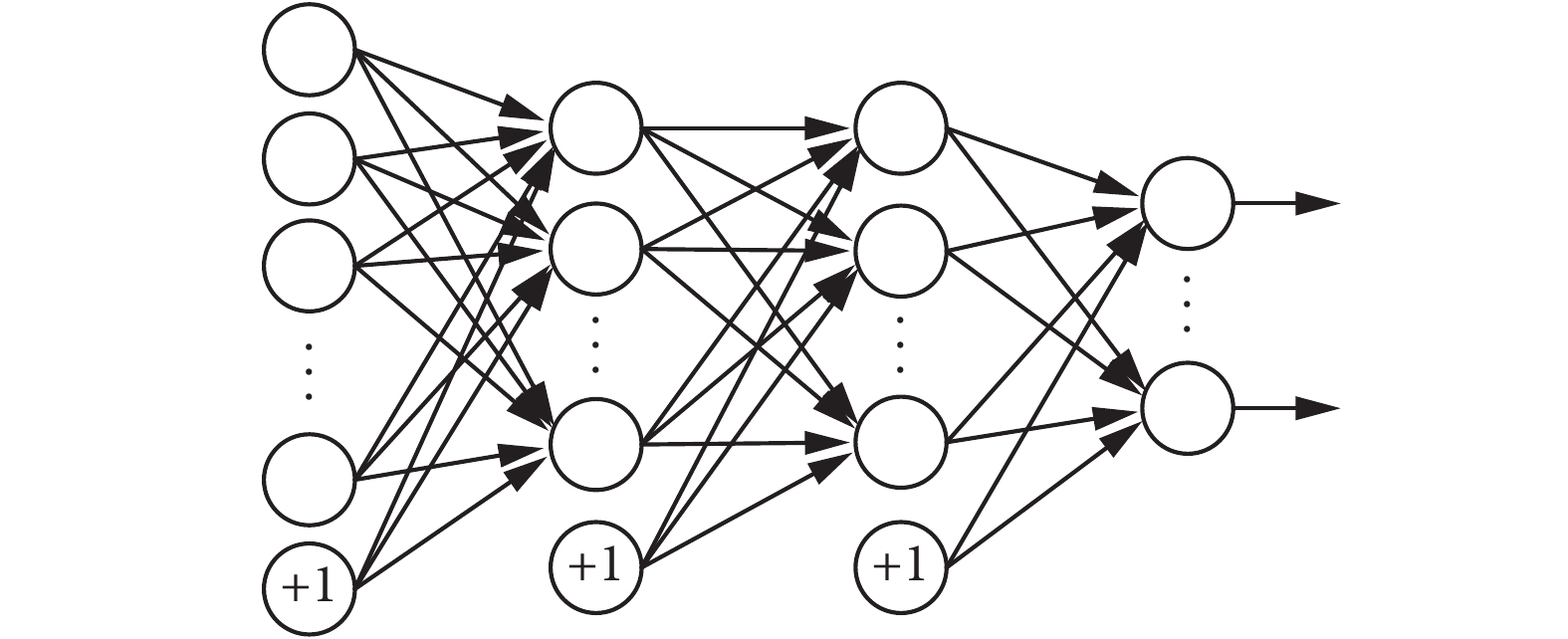图 2 栈式自编码器示意图 Fig.2 Stacked auto-encoder diagram
3 特征迁移模型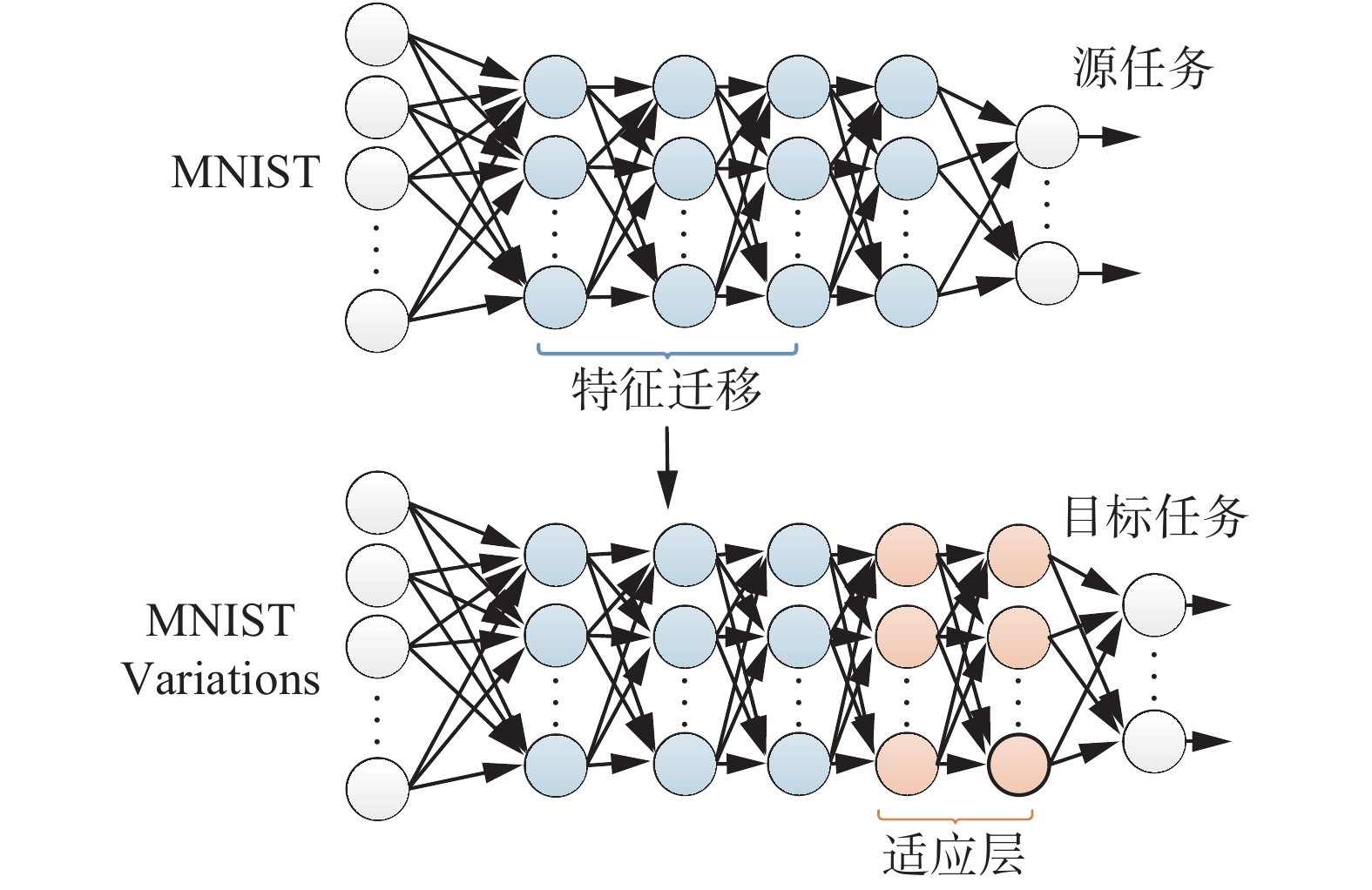图 3 特征迁移模型示意图 Fig.3 Feature transfer model diagram

 ${{\mathit{\boldsymbol{z}}}^{(l + 1)}} = {{\mathit{\boldsymbol{W}}}^{(l)}}{{\mathit{\boldsymbol{a}}}^{(l)}} + {{\mathit{\boldsymbol{b}}}^{(l)}}$
 ${{\mathit{\boldsymbol{a}}}^{(l + 1)}} = f\left( {{\mathit{\boldsymbol{z}}}^{(l + 1)}} \right)$

 ${h_{\mathit{\boldsymbol{\theta }}}}({\mathit{\boldsymbol{x}}}) = \left[ {\begin{array}{*{20}{c}}{p(y = 1|{\mathit{\boldsymbol{x}}};{\mathit{\boldsymbol{\theta }}})}\\ \vdots \\{p(y = k|{\mathit{\boldsymbol{x}}};{\mathit{\boldsymbol{\theta }}})}\end{array}} \right] = \frac{1}{{\sum\nolimits_{i = 1}^k {{{\rm{e}}^{{\mathit{\boldsymbol{\theta}}}_i^{\rm{T}}{\mathit{\boldsymbol{x}}}}}} }}\left[ {\begin{array}{*{20}{c}}{{{\rm{e}}^{{\mathit{\boldsymbol{\theta}}}_1^{\rm{T}}{\mathit{\boldsymbol{x}}}}}}\\ \vdots \\{{{\rm{e}}^{{\mathit{\boldsymbol{\theta}}} _k^{\rm{T}}{\mathit{\boldsymbol{x}}}}}}\end{array}} \right]$

 $J({\mathit{\boldsymbol{\theta }}}) = - \frac{1}{m}\sum\nolimits_{i = 1}^m {\sum\nolimits_{j = 1}^k {1\left\{ {{y^{(i)}} = j} \right\}} } \log \frac{{{{\rm{e}}^{{\mathit{\boldsymbol{\theta}}} _l^{\rm{T}}{{\mathit{\boldsymbol{x}}}^{(i)}}}}}}{{\sum\nolimits_{l = 1}^k {{{\rm{e}}^{{\mathit{\boldsymbol{\theta}}} _l^{\rm{T}}{{\mathit{\boldsymbol{x}}}^{(i)}}}}} }}$

 $1\left\{ {{y^{(i)}} = j} \right\}{\rm{ = }}\left\{ {\begin{array}{*{20}{c}}{1,} &\quad {{y^{(i)}} = j}\\{0,} &\quad {{y^{(i)}} \ne j}\end{array}} \right.$
4 实验比较

MNIST数据集由0～9的手写体数字组成，10种数字构成了10类数据。MNIST包含60 000张训练图像和10 000张测试图像。图4给出了MNIST数据集的一些示例样本。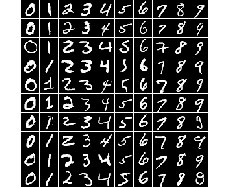图 4 MNIST示例样本 Fig.4 MNIST samples

MNIST variations包含MNIST的4种变体数据集，包括：MNIST-rot，即在手写体数字上施加一些随机旋转；MNIST-back-rand，即在数字图像中插入随机的背景；MNIST-back-image，一小块黑白图像被用作数字图像的背景；MNIST-rot-back-image，即MNIST-rot与MNIST-back-image相结合。图5给出了MNIST-variations数据集的一些示例样本。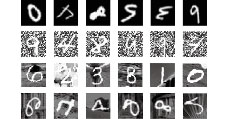图 5 MNIST-variations示例样本 Fig.5 MNIST-variations samples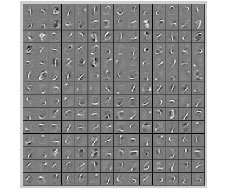图 6 MNIST中级特征 Fig.6 MNIST mid-level features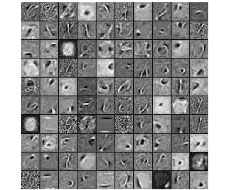图 7 MNIST-variations中级特征 Fig.7 MNIST-variations mid-level features表 1 分类错误率 Tab.1 Classification error rate
5 结束语

  HINTON G E, ZEMEL R S. Autoencoder minimum description length and helmholtz free energy[C]//Conference on Neural Information Processing Systems(NIPS). Denver, USA, 1993: 3–10. (0)  SOCHER R, HUANG E H, PENNINGTON J, et al. Dynamic pooling and unfolding recursive autoencoders for paraphrase detection[C]//Proc Neural Information and Processing Systems. Granada, Spain, 2011: 801–809. (0)  SWERSKY K, RANZATO M, BUCHMAN D, et al. On score matching for energy based models: generalizing autoencoders and simplifying deep learning[C]//Proc Int’l Conf Machine Learning Bellevue. Washington, USA, 2011: 1201–1208. (0)  FISCHER A, IGEL C. An introduction to restricted Boltzmann machines[C]//Progress in Pattern Recognition, Image Analysis, Computer Vision, and Applications. Guadalajara, Mexico, 2012: 14–36. (0)  BREULEUX O, BENGIO Y, VINCENT P. Quickly generating representative samples from an RBM-derived process[J]. Neural computation, 2011, 23(8): 2053-2073. (0)  COURVILLE A, BERGSTRA J, BENGIO Y. Unsupervised models of images by spike-and-slab RBMs[C]//Proc Int’l Conf Machine Learning. Bellevue, Washington, USA, 2011: 1145–1152. (0)  SCHMAH T, HINTON G E, ZEMEL R, et al. Generative versus discriminative training of RBMs for classification of fMRI images[C]//Proc Neural Information and Processing Systems. Vancouver, Canada, 2008: 1409–1416. (0)  ERHAN D, BENGIO Y, COURVILLE A, et al. Why does unsupervised pre-training help deep learning?[J]. Machine learning research, 2010, 11: 625-660. (0)  VINCENT P. Extracting and composing robust features with denoising auto-encoders[C]//International Conference on Machine Learning(ICML). Helsinki, Finland, 2008: 1096–1103. (0)  VINCENT P, LAROCHELLE H, LAJOIE I, et al. Stacked denoising autoencoders: learning useful representations in a deep network with a local denoising criterion[J]. Machine learning research, 2010, 11: 3371-3408. (0)  CHEN M, XU Z, WINBERGER K Q, et al. Marginalized denoising autoencoders for domain adaptation[C]//International Conference on Machine Learning. Edinburgh, Scotland, 2012: 1206–1214. (0)  VINCENT P. A connection between score matching and denoising autoencoders[J]. Neural computation, 2011, 23(7): 1661-1674. (0)  RIFAI S. Contractive auto-encoders: explicit invariance during feature extraction[C]//Proceedings of the Twenty-eight International Conference on Machine Learning. Bellevue, USA, 2011: 833–840 (0)  RIFAI S, BENGIO Y, DAUPHIN Y, et al. A generative process for sampling contractive auto-encoders[C]//Proc Int’l Conf Machine Learning. Edinburgh, Scotland, UK, 2012: 1206–1214. (0)  LECUN Y. Neural networks: tricks of the trade (2nd ed.) [M]. Germany: Springer, 2012: 9–48 (0)  ZEILER M D, TAYLOR G W, FERGUS R. Adaptive deconvolutional networks for mid and high level feature learning[C]//IEEE International Conference on Computer Vision. Barcelona, Spain, 2011: 2013–2025. (0)